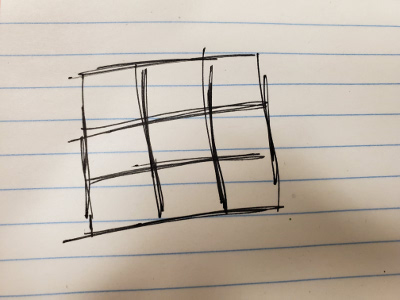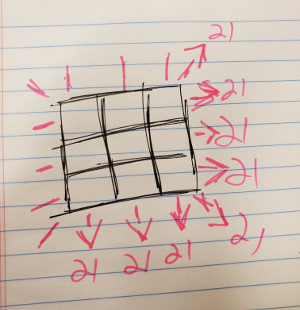## The Problem

This is a logic problem from my son’s homework in middle school. We start with a 3x3 square, with nine cells.We are then given these numbers: `3, 4, 5, 6, 7, 8, 9, 10, 11`. What we want is to place them within the square such that the sum of every row, every column and every diagonal is `21`.There is a key insight where, once you get it, the solution just falls into place. But, I’m a programmer, and a programmer looks at this and sees brute force solutions, so I wrote one. And I pulled another language and did it again and again.

## First JS Solution

For reasons, I started looking at Rust, but I’m not far enough along to implement this in Rust, so I pulled out a language I’ve been playing with a lot recently, Javascript.

And, as it turns out, that was a first-pass language for this as well. Here’s my old code:

``````function main() {
var numbers = range(3, 11);
var array = [];
recurse_magic_box(numbers, array);
}

function recurse_magic_box(numbers, array) {
for (var n in numbers) {
var num = numbers[n];
array.push(num);
if (check_magic_box(array)) {
recurse_magic_box(numbers, array);
}
array.pop();
}
}

function check_magic_box(array) {
if (contains_duplicates(array)) {
return 0;
}
if (array.length > 9) {
return 0;
}
if (array.length == 9) {
var sum = 21;
if (sum != array + array + array) {
return 0;
}
if (sum != array + array + array) {
return 0;
}
if (sum != array + array + array) {
return 0;
}
if (sum != array + array + array) {
return 0;
}
if (sum != array + array + array) {
return 0;
}
if (sum != array + array + array) {
return 0;
}
if (sum != array + array + array) {
return 0;
}
if (sum != array + array + array) {
return 0;
}
console.log(["[", array, array, array, "]"].join(" "));
console.log(["[", array, array, array, "]"].join(" "));
console.log(["[", array, array, array, "]"].join(" "));
console.log("");
}
return 1;
}

function contains_duplicates(array) {
var check = [];
for (var i = 0; i < array.length; i++) {
var n = array[i];
if (check[n] == 1) {
return 1;
}
check[n] = 1;
}
return 0;
}

function range(start, end) {
return Array(++end - start)
.join(0)
.split(0)
.map(function(n, i) {
return i + start;
});
}

main();
``````

This is a bit more verbose than I like, but it is working code. I have been playing with `map()`, `reduce()` and arrow functions recently, so how would I do this now?

## Modern JS Solution

``````"use strict";

recursive_magic_box(
[],
Array(9)
.fill()
.map((v, i) => 3 + i)
);

function recursive_magic_box(array, numbers) {
for (let i in numbers) {
let n = numbers[i];
array.push(n);
if (check(array)) {
recursive_magic_box(array, numbers);
}
array.pop();
}
}

function check(array) {
let flag = 1;
let sum = 21;
let checks = [
[0, 1, 2],
[3, 4, 5],
[6, 7, 8],
[0, 3, 6],
[1, 4, 7],
[2, 5, 8],
[0, 4, 8],
[2, 4, 6]
];
array.map((v, i, a) => {
let j = a.indexOf(v);
let k = i === j ? 1 : 0;
if (!k) {
flag = 0;
}
});
if (flag === 0) return 0;
if (array.length == 9) {
for (let c in checks) {
let d = checks[c].map(v => array[v]).reduce((s, v) => (s += v));
if (d != sum) {
return 0;
}
}
display(array);
}
return 1;
}

function display(array) {
for (let i = 0; i < 9; i += 3) {
console.log(
Array(3)
.fill()
.map((v, j) => array[j + i])
);
}
console.log();
}
``````

Some of the changes: I don’t have a `range` function, instead using `Array(9).fill().map((v, i) => 3 + i)` to give me `3..11`. I stay with a C-style `for` loop in `recursive_magic_box()`, which could’ve gone the other way. I was killing time, coding from my phone before an event, and that made that choice.

I think the changes in `check()` are the most telling. We are checking a few things: if we’re repeating a digit, if we’re at 9 digits in the array, and if all the diagonals are correct. In testing, I could get returning from within the `map` to work, so I set a flag and return 0 if the flag is 0.

In `let k = i === j ? 1 : 0`, I use a ternary because I see these return `1` or `null` rather than `0`, and that breaks the logic in ways that suprise me. Might not be necessary, but that’s what I do.

The way I do checks for `21`, however, is the coolest part. I start with the array of arrays, because we’re working with `[0,1,2,3,4,5,6,7,8,9]` but thinking `[[0,1,2],[3,4,5],[6,7,8]]`. The array of arrays allows us to not have the series of `else if` statements.

Instead, we have `let d = checks[c].map(v => array[v]).reduce((s, v) => (s += v))`, which carries a lot of freight. Let us assume we’re trying to test `[3,4,5,6,7,8,9,10,11]`. `c` is an index; when `c==0`, `checks[c] = [0,1,2]`. `map(v => array[v])` uses `[0,1,2]` as the positions within `array` and gives use `[3,4,5]`.

We don’t have `Math.sum()` in JS, but we do have `reduce`. It takes four values: what we’re writing to, the current value, the current index, and the array we’re working with, but we only need the first two to make sum. `reduce((s,v) => (s+=v))` adds the current value to s, then returns the single value `12`, which clearly is not `21`, so we return zero and go on.

I also use loopy cleverness to print the output. The all-in-one solution would be `Array(3).fill(0).map((v,i)=>Array(3).fill().map((x,y)=>array[y+(i*3)]))` but I didn’t have that figured out before I started writing this.

If you have any questions or comments, I would be glad to hear it. Ask me on Twitter or make an issue on my blog repo.